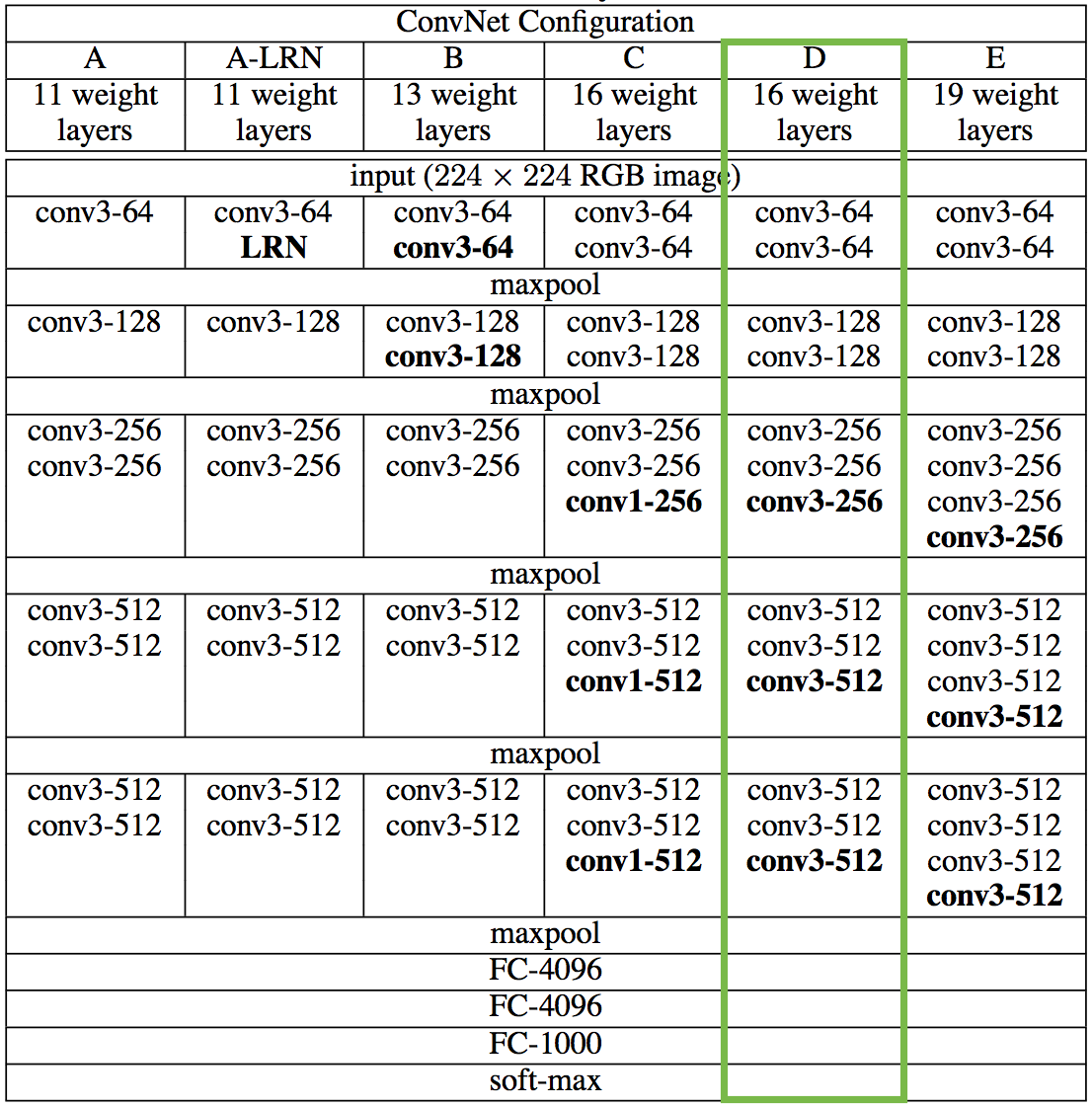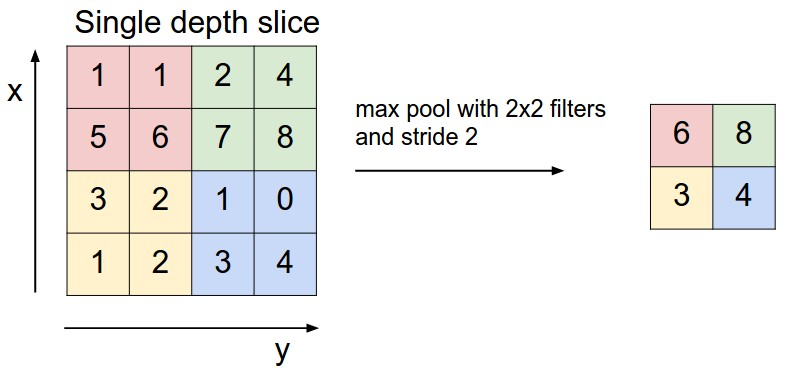Build VGG16 from scratch: Part I

In the two first posts we used a pre-trained model VGG16. VGG16 is a convolutional neural network (CNN) containing only 16 weight layers. Because it has a simple architecture we can build it conveniently from scratch with Keras.

This article will refer regularly to the original paper of VGG networks. The purpose of this first part is to explain the functions of the layers of a CNN. It is also an opportunity to show how simple are the function of each layer, that’s why I will implement each of these methods in Python without Keras.

1 . The VGG16 architecture

The VGG16 architecture is the one in green : it contains 16 weight layers (13 convolutional layers and 3 fully connected layers). Note that the ReLU layers are not mentioned in this first illustration.The VGG architecture from the original article (the ReLU layer are not represented)VGG16 architecture with layers sizes from this post (with the ReLU)

With Keras we can have the layers and their shapes :

_________________________________________________________________
Layer (type)                 Output Shape              Param #
=================================================================
input_1 (InputLayer)         (None, 224, 224, 3)       0
_________________________________________________________________
block1_conv1 (Conv2D)        (None, 224, 224, 64)      1792
_________________________________________________________________
block1_conv2 (Conv2D)        (None, 224, 224, 64)      36928
_________________________________________________________________
block1_pool (MaxPooling2D)   (None, 112, 112, 64)      0
_________________________________________________________________
block2_conv1 (Conv2D)        (None, 112, 112, 128)     73856
_________________________________________________________________
block2_conv2 (Conv2D)        (None, 112, 112, 128)     147584
_________________________________________________________________
block2_pool (MaxPooling2D)   (None, 56, 56, 128)       0
_________________________________________________________________
block3_conv1 (Conv2D)        (None, 56, 56, 256)       295168
_________________________________________________________________
block3_conv2 (Conv2D)        (None, 56, 56, 256)       590080
_________________________________________________________________
block3_conv3 (Conv2D)        (None, 56, 56, 256)       590080
_________________________________________________________________
block3_pool (MaxPooling2D)   (None, 28, 28, 256)       0
_________________________________________________________________
block4_conv1 (Conv2D)        (None, 28, 28, 512)       1180160
_________________________________________________________________
block4_conv2 (Conv2D)        (None, 28, 28, 512)       2359808
_________________________________________________________________
block4_conv3 (Conv2D)        (None, 28, 28, 512)       2359808
_________________________________________________________________
block4_pool (MaxPooling2D)   (None, 14, 14, 512)       0
_________________________________________________________________
block5_conv1 (Conv2D)        (None, 14, 14, 512)       2359808
_________________________________________________________________
block5_conv2 (Conv2D)        (None, 14, 14, 512)       2359808
_________________________________________________________________
block5_conv3 (Conv2D)        (None, 14, 14, 512)       2359808
_________________________________________________________________
block5_pool (MaxPooling2D)   (None, 7, 7, 512)         0
_________________________________________________________________
flatten (Flatten)            (None, 25088)             0
_________________________________________________________________
fc1 (Dense)                  (None, 4096)              102764544
_________________________________________________________________
fc2 (Dense)                  (None, 4096)              16781312
_________________________________________________________________
predictions (Dense)          (None, 1000)              4097000
=================================================================

Total params: 138,357,544
Trainable params: 138,357,544
Non-trainable params: 0
_________________________________________________________________

2 . Layers

A. Input layer

We start with the basics : the input layer. It is the image we want to classify with a bit of preprocessing : “The only preprocessing we do is subtracting the mean RGB value, computed on the training set, from each pixel.”.

The input is a 224 x 224 BGR image, that’s why the input shape is (224,224,3). The3 represent the three color channel in the image. The preprocessing consists of three steps : resize the image, subtract the mean of the trained data and convert the RGB image to a BGR image.

B. Convolutional layers

After this input layer there is a stack of convolutional layers in the VGG16 model. What are these convolutional layers ?

The convolution layers take patches of n*n pixels and apply a matrix multiplication. Then the sum of the multiplication become a new pixel. Note that each patch is multiplied by the same matrix.

For example in this GIF the weight matrix is W = [[0,1,2],[2,2,0],[0,1,2]] . For each patch we take the sum of the multiplication of the patch by the matrix. The input matrix is 5×5 and the output 3×3source

Now lets apply the convolution process on an image to understand its effects.our original image

Depending on the weight we can see that the output will spotlight different features of the image.More on the convolutional layers :

C. ReLU – activation function

In the VGG16 model each convolutional layer is followed by a ReLU layer which is an activation function. ReLu is a non linear function : f(x) = max(0,x). Without these activation layers the stack of convolutional layers (which are linear) could be simplify to one linear calculus and it would be equivalent at a single layer.

x = [[-0.49835121 -0.27024171 -0.00921487]
[-0.222737    0.2307323  -0.14144912]]
relu(x) = [[ 0.         0.         0.       ]
[ 0.         0.2307323  0.       ]]

Did you note how simple this function is ? It can be implemented in one line of Python !

D. Max Pooling

In the VGG16 architecture we saw that after each two or three convolutional layers there was a max pooling layer. The purpose of this layer is to reduce the shape of the arrays. It will also prevent overfitting.

For each patch of n*n pixels, it takes the biggest one and it replaces the entire patch. For exemple on this example : the 4×4 matrix become a 2×2 matrix after max pooling.With the block_reduce function of skimage this layer can be implemented in one line of python.

x = [[1 4 4 1 2 2]
[0 4 1 2 4 2]
[3 1 0 3 3 0]
[2 0 3 1 3 4]
[0 0 4 0 1 1]
[2 0 3 1 2 1]]

max_pooling(x) = [[4 4 4]
[3 3 4]
[2 4 2]]

Max-pooling requires an parameter called “strides”. In these examples the strides has the value of the square lengths. It defines how much the first square is moving before it choose the max. If it’s unclear, there is a good visual explanation here.

E. Dense or fully-connected

After the stack of conv layers there are three fully-connected layers. The function behind a FC layer is a linear operation where each input is multiply by a specific weight. It is simply a matrix multiplication, that’s why is should be followed by an activation function (a ReLU for VGG16).

F. Softmax – activation function

The last layer of the VGG16 is a softmax function. It is simply the exponential of each input divided by the sum of the exponential. It results in a vector of length 1000 where each scalar is the probability to belong to one of the 1000 category of ImageNet. Note that the sum of the output is 1, and that is what we want because in ImageNet the category are exclusive).x = [ 8 14 16  8 14  1]

softmax(x) = [  2.63865019e-04   1.06450746e-01   7.86570537e-01
2.63865019e-04  1.06450746e-01   2.40613752e-07]

Conclusion

In this first part we have explain the part of each layer in VGG16 and I have show you that it is really simple function from a mathematical and from a programming point of view. In the second part of this article on VGG16 we will implement the network with Keras.